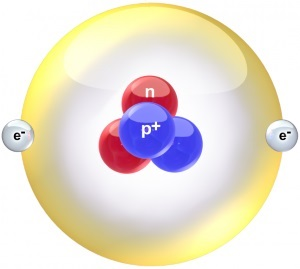#Basic Electronics for Computer Architecture by Stephen Bucaro

To understand computer architecture, it is necessary for you to have a basic understanding of electronics. All the amazing things that can be done with electronics are the result of one simple thing - electric charge. Electric charge comes in two forms, positive and negative. A positive charge is the electrostatic field radiated by protons. A negative charge is the electrostatic field radiated by electrons.

The basic construction of an atom, the particle of which all matter is made, is a central nucleus containing protons surrounded by outer shells created by electrons orbiting the nucleus. The power that comes from electricity is caused by the fact that same charges repel each other and opposite charges attract each other. In other words, two protons repel each other, two electrons repel each other, but an electron and a proton attract each other.Helium atom
Source: Wikipedia Commons/BruceBlaus

The nucleus of an atom can contain many positively charged protons, along with many neutrally charged particles called neutrons. You might ask, "if same charges repel each other, how does the nucleus stay together?" The repulse force of the protons in the nucleus of an atom is overcome by a nuclear force called the "strong force". Interestingly, there is another force acting in the nucleus of an atom called the "weak force" that eventually overcomes the strong force, allowing protons to leave the nucleus. The weak force is responsible for radioactive decay.

The strong force and the weak force in the nucleus of an atom is caused by quarks. A quark is a sub-atomic particle of which protons are constructed. But in our effort to learn basic electronics, we don't want to delve too deeply into nuclear physics, so let's just stick with the fact that atoms are constructed of a nucleus containing positively charged protons and neutrally charged neutrons, and the nucleus is surrounded by shells of orbiting negatively charged electrons. Sometimes the shell of orbiting electrons is referred to as an "electron cloud".

One important fact is that the nucleus of an atom is relatively heavy. That's because a proton is about 1800 times more massive than an electron. The reason this is important is because electricity primarily involves the flow of electrons. The nucleus of an atom generally doesn't move much. The atoms of some elements hold onto their electrons tightly. These elements are called "insulators".

The atoms of some elements, like copper and aluminum, hold onto their electrons loosely, especially the electrons that orbit in the outer shells. It is these electrons that tend to break loose and can be used to create electron flow. Elements that hold onto their electrons loosely are called "conductors".

There is a third type of material, elements like germanium and silicon, that sometimes conduct and sometimes don't, and sometimes conduct some but not fully. It depends on how you treat, or "bias" them. These materials are called semiconductors and they are responsible for much of our modern electronics. But we won't delve into semiconductors in this basic introduction.

There are two basic types of electron flow - direct current (DC) and alternating current (AC). DC current flows in one direction, from a more negatively charged location to a less negatively charged location. Since same charges repel each other, negatively charged electrons will flow in an attempt to get as far away from each other as possible.

With AC current, the flow of charge reverses direction periodically. The rate of this reversal is measured in cycles per second, a unit referred to as Hertz (Hz). The AC power used in a house has a reversal rate, or frequency, of 50 Hz.

The amount of current flowing in a circuit is measured in Amperes (Amps). If you were comparing electron flow to water flow, you might compare amps to gallons. The strength of charge in a circuit is measured in Volts (V). You might compare volts, or voltage, to the pressure of water coming out of a faucet or hose. In AC, the voltage polarity reverses along with the direction of current flow.

One strange thing in electronics is that, although electrons flow from a more negatively charged location to a less negatively charged location, in other words from negative to positive, technicians and engineers always consider current to be flowing from positive to negative. This is because when Benjamin Franklin experimented with electricity, he assumed that the electrical current must be positive. We now understand that electrons have a negative charge, and that the flow of electrons is actually in the opposite direction.

Usually positive is the power source labeled Vcc, and the current is visualized to be flowing to Ground (Gnd), which is defined as zero volts. Strangely enough, this reverse thinking doesn't usually cause any problems in electronic calculations.

One last thing needs to be covered in this basic introduction to electronics. In electronic circuits, there is a resistance to the flow of current. This resistance to the flow of current is appropriately called "Resistance" and it's measured in a unit called "Ohms". There is an equation commonly used in electronics called "Ohm's law" that says E = I x R (volts equals amps times ohms). Rearranging this formula allows you to calculate almost everything you might want to know about a DC circuit.

There is another type of "resistance" in AC circuits called "reactance", but we don't want to get involved in that in this basic introduction.